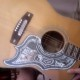### the algebraization of the rainbow (remix)i’ve given the equations of the (seven)
planes-in-ordinary-(x, y, z)-space
that pass through the various
“color triples” of rainbow space—
the blends, the blurs, and the ideal.

(upon putting the “primaries” [*not* one
of our triples] {R, Y, B} onto the vertices
(100, 010, and 001) of a cube
[namely, the “unit cube” I^3=$\{ (x,y,z) | 0 \le x,y,z \le 1 \}$
if you wanna get all *technical*…].)

somewhat awkwardly, *one* of our planes
(up in the upper right somewhere) does
*not* pass through (0,0,0)
(or 000 as it’s also called here).

what gives?

mod 2 arithmetic, is what.
the equations in the display “work”
in good old-fashioned${\Bbb R}^3$
or E^3(R) [euclidean 3-space]—
but to get the 7-point-space
— P^2 (F_2) [projective 2-space
over the 2-element field]… if
you wanna get all *technical*…—
we have to “work mod 2”.

and, believe it or not, 0 = 2
on this model. (so we pick up
(0, 0, 0) as a solution to
“x+y+z = 2”
[which it now becomes more
convenient to write as
“x + y + z = 0 (mod 2)”
]).

and that’s essentially it.

this “dualization” i’ve been
going on about for years, now?
here it is.
the set-of-seven *equations*
(
or their planes in three-space,
or their color-triples,
or their color-triples-plus-000,
or the “lines” of P^2(F_2)…
these are all ways of saying
the same thing…
)
can *also* be given a 7-point
“fano space” structure.

and has been (in some sense),
here on the page, via the [X:Y:Z] notation.

(note that the set-of-seven *points*
[i.e., any two distinct points
determine a unique 3-point line]).

(details suppressed with great effort…
part of the point here is that we
*don’t* need the [“linear algebra”]
formalism [usually learned, oddly
enough, in “calculus” classes (if at
all)]—“dot products” and so on—
to achieve our dualization: we
can just *draw* the doggone thing
and check directly that our structure
“works”.)

thank you and good day.

1.vlorbik
2.vlorbik

https://vlorbik.wordpress.com/2013/02/17/tear-down-the-wall/
(of course the whole mess embeds into 21-point space.
but for *that*, i still need the algebra.)

3.vlorbik
1. 1 Carnival Of Mathematics #122 | Solve My Maths

[…] Vlorbik discusses the algebraization of the rainbow […]

• ## (Partial) Contents Page

Vlorbik On Math Ed ('07—'09)
(a good place to start!)